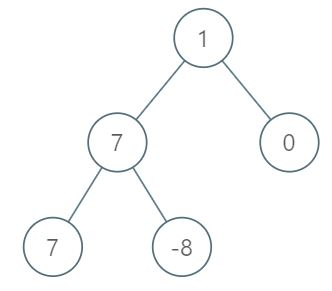# 1161. Maximum Level Sum of a Binary Tree

Medium
Given the `root` of a binary tree, the level of its root is `1`, the level of its children is `2`, and so on.
Return the smallest level `x` such that the sum of all the values of nodes at level `x` is maximal.
Example 1:Input: root = [1,7,0,7,-8,null,null]
Output:
2
Explanation:
Level 1 sum = 1.
Level 2 sum = 7 + 0 = 7.
Level 3 sum = 7 + -8 = -1.
So we return the level with the maximum sum which is level 2.
Example 2:
Input: root = [989,null,10250,98693,-89388,null,null,null,-32127]
Output:
2
Constraints:
• The number of nodes in the tree is in the range `[1, 104]`.
• `-105 <= Node.val <= 105`

### 解題

Runtime: 143 ms, faster than 84.33%
Memory Usage: 8.7 MB, less than 55.56%
/**
* Definition for a binary tree node.
* type TreeNode struct {
* Val int
* Left *TreeNode
* Right *TreeNode
* }
*/
func maxLevelSum(root *TreeNode) int {
m := make(map[int]int)
var helper func(*TreeNode, int)
helper = func(root *TreeNode, depth int) {
if root == nil {
return
}
if _, ok := m[depth]; ok {
m[depth] += root.Val
} else {
m[depth] = root.Val
}
helper(root.Left, depth + 1)
helper(root.Right, depth + 1)
}
helper(root, 1)
max := 1
for k := range m {
if m[k] > m[max] {
max = k
}
}
return max
}